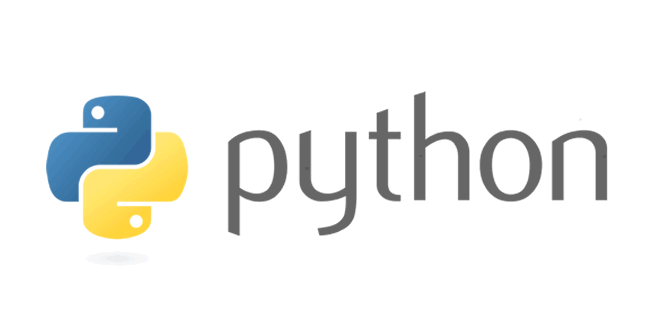Tuesday , August 9 2022# 12th Class Computer Science Pre-Mid Exam 2022

 School Name: Himalaya Public School, Sector 13, Rohini, Delhi 110085 India Class: 12th Standard (CBSE) Subject: Computer Science Time Duration: 01 Hours Maximum Marks: 30 Date: 04 / 07 / 2022

### General Instructions: 12th Class Computer Science Pre-Mid Exam 2022-23

• All questions are compulsory
• Give examples wherever possible

#### Question 02: Find the error (if any) in the following code and write the corrected code and underline it: (2)

return a+b:
print( “The sum =” Sum(7,-1)

#### Question 03 – 12th Computer Science Exam: State the difference between: (4)

1. Built in functions and functions using libraries
2. Default argument and positional arguments.

#### Question 04: Identify the type one or types of arguments in the following codes: (3)

a) def sum(a=1,b):
return a+b
print(sum(b=20, a=5 ))

b) def sum(a=1,b):
return a+b
print (sum(10,20))

#### Question 08: Write a program using user defined function to:

1. Check if a number entered by the user to check whether a number is even or not also if it is divisible by 6 or not. (3)
2. Calculate and display average of all the elements in a user defined tuple containing numbers. (4)

#### Question 09: Write the output of the following statements: (4)

• sring1=’Computer Science’
print(string1.isupper())
• import math as m
print(m.ceil(15.890))
• stringl=’Tonight’
print(len(stringl))
• import math as m
print(m.pow(5,2))